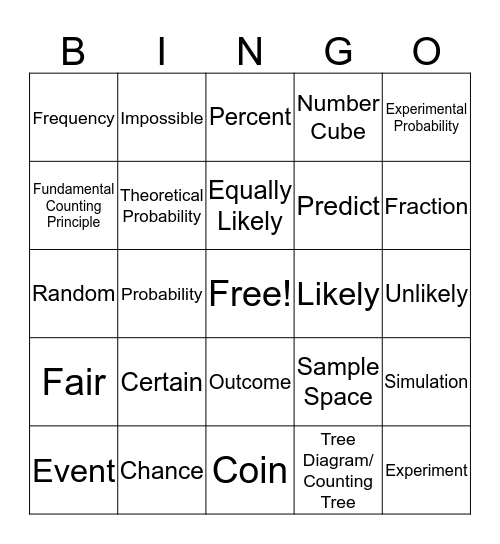Probability VocabularyThis bingo card has a free space and 24 words: Certain, Chance, Equally Likely, Event, Experimental Probability, Frequency, Fundamental Counting Principle, Impossible, Likely, Outcome, Predict, Random, Sample Space, Simulation, Theoretical Probability, Tree Diagram/ Counting Tree, Unlikely, Percent, Fraction, Probability, Experiment, Fair, Number Cube and Coin.

Play Online